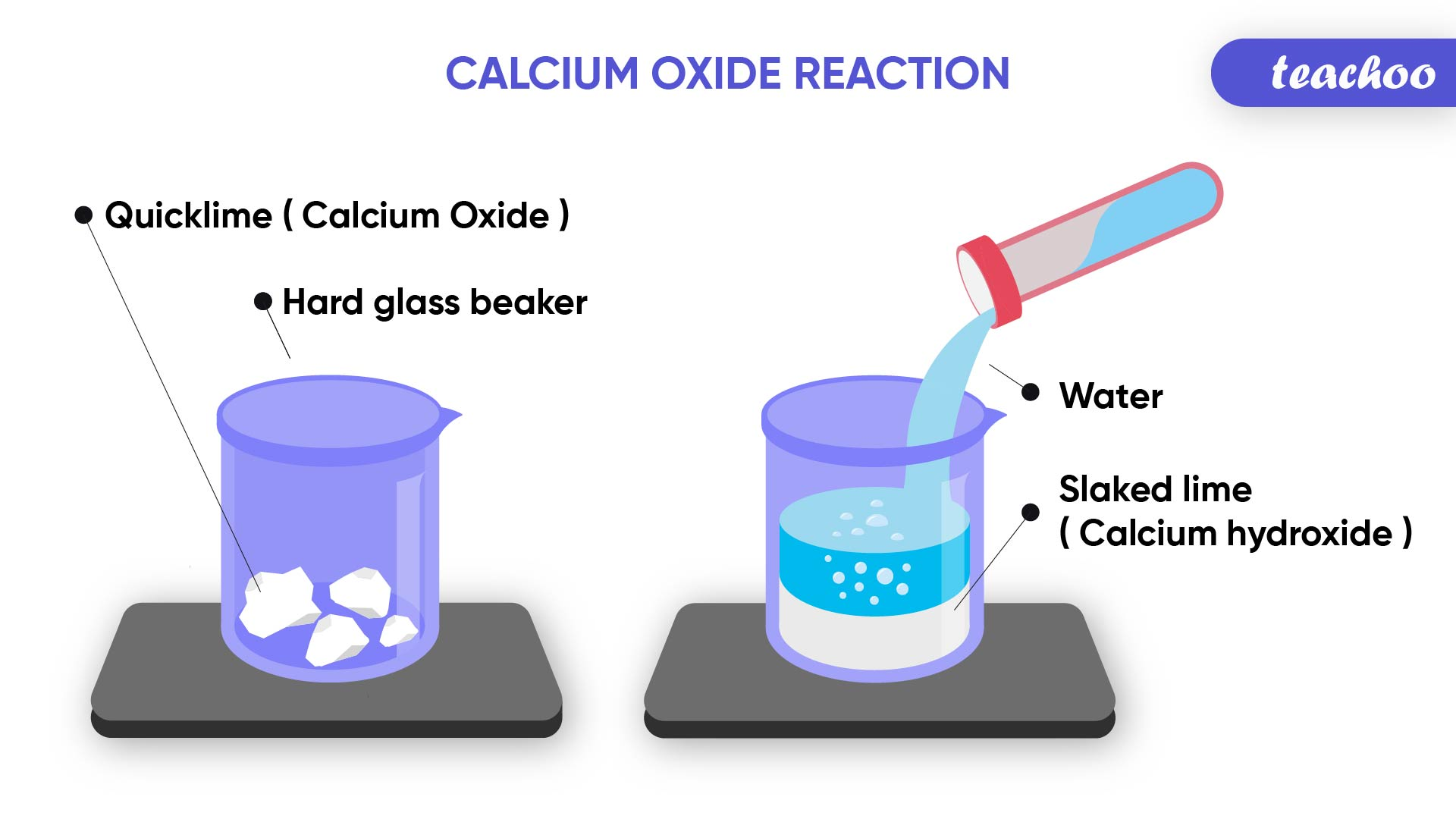Concepts

Class 10
Chapter 1 Class 10 - Chemical Reactions and Equations

When two or more substances react to form a single product , the reaction is called combination reaction .

## Example 1

Production of Slaked Lime

Calcium Oxide (Quick Lime) reacts with Water to produce Calcium Hydroxide (Slaked Lime) and Heat

CaO + H 2 O → Ca(OH) 2In this case, there are 2 reactants,

1. Calcium Oxide(CaO) and
2. Water (H 2 O)

They produce single product - Calcium Hydroxide - Ca(OH) 2 (Slaked Lime)

## What is quick lime and slaked lime?

Calcium Oxide (CaO) is known as quick lime .

It is used for manufacturing cement .

Calcium Hydroxide (Ca(OH) 2 ) is known as slaked lime .

Slaked lime is formed by the reaction of quick lime and water by the following reaction.

CaO(s) + H 2 O(l) → Ca(OH) 2 (aq)

This above reaction forms an aqueous solution of slaked lime .

This is used for whitewashing .

The Calcium Hydroxide (Ca(OH) 2 ) reacts with CO 2 present in the air to form a thin layer of Calcium Carbonate (CaCO 3 ) on the walls.

The reaction is as follows

Ca(OH) 2 + CO 2 → CaCO 3 + H 2 O

CaCO 3 is formed after 2-3 days of whitewashing .

It gives a shiny finish to the walls.

Chemical formula for marble is also CaCO 3  .

## Example 2

Burning of coal

Coal burns in oxygen to produce Carbon Dioxide.

Again 2 reactants produce 1 product.

C(s) + O 2 (g) → CO 2 (g)

## Example 3

Formation of Water

Hydrogen and Oxygen combine to form water.

2H 2 (g) + O 2 (g) → 2H 2 O(l)

## What are Exothermic Reactions?

A chemical reaction in which a large amount of heat/energy is produced along with the products is called Exothermic Reaction.

## Example 1

Production of Slaked Lime

Calcium Oxide (Quick Lime) reacts with Water to produce Calcium Hydroxide (Slaked Lime) and Heat

CaO(s) + H 2 O(l) → Ca(OH) 2 (aq)

Since heat is produced in this reaction, this reaction is called an exothermic reaction.

The above reaction is an example of both combination reaction and exothermic reaction.

## Example 2

Burning of Natural Gas

CH 4 (Methane) is known as natural gas

CH 4 (g) + 2O 2 (g) → CO 2 (g) + 2H 2 O (g)

In this reaction, a large amount of heat is produced, hence it is a exothermic reaction

However, this is not a combination reaction as more than 1 product is produced.

## Example 3

Decomposition of Vegetable matter into compost

In this reaction, a large amount of heat is produced, hence it is an exothermic reaction.

## Example 4

Respiration is also an exothermic process.

What is respiration?

During digestion, food is broken down into simpler substances.

Rice, bread, potatoes etc. contain carbohydrates which are broken down into their simple form; glucose.

Glucose combines with the oxygen present in our body and provides energy .

This is known as respiration .

C 6 H 12 O 6 (aq) + 6O 2 (aq) → 6CO 2 (aq) + 6H 2 O(l) + energy

(Glucose)

Since it involves release of energy, it is an exothermic process.

Intext Question - Page 10 Q1

NCERT Exercise - Q10

Introducing your new favourite teacher - Teachoo Black, at only ₹83 per month